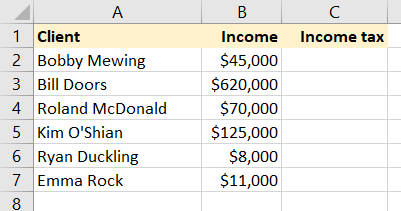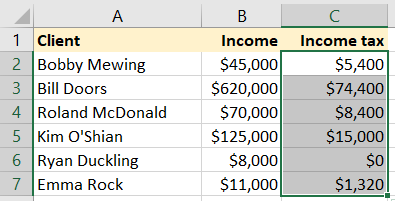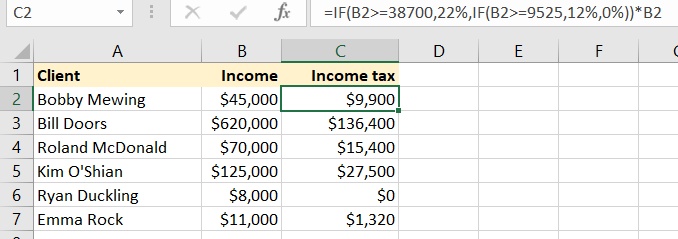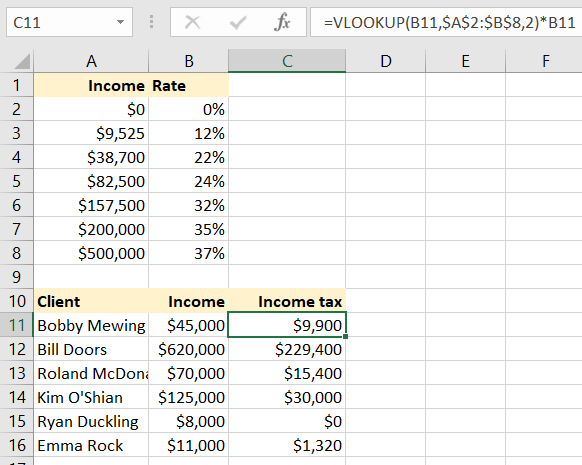Voices

# The most useful Excel formulas for accountants

Register now

Microsoft Excel was first released in 1987 and — despite popular competitors such as Google Sheets — is still used by millions of businesses throughout the world. Described as the “world’s most popular productivity tool,” research suggests that almost 90 percent of companies use Excel for budgeting, planning and forecasting operations.

Given the nature of our business, perhaps it is no surprise that this software is so popular. During the first stages of accountancy training, it is likely you used Excel to initially keep track of figures. As a familiar product, it makes sense that you would carry it over into your day-to-day work.

Although you might view yourself as an Excel expert, there is always room for improvement. Potentially, you could save hours of time with just a few formulas. Excel training experts Wise Owl shows two functions that can make your working life easier: IF and VLOOKUP.

Conditional IF Formula

The =IF function in Excel allows you to test whether a condition is true or not, and returns different results accordingly. The syntax of this handy little function is as follows:

=IF ( condition to test, what to do if it’s true, what to do if it’s false )

For example, suppose you are an accountant trying to work the US income tax for 2018 for a series of (unmarried) clients:You want to find out the income tax that each client would pay. Let’s suppose a simple world in which people pay no income tax up to \$9,525, and income tax at 12% thereafter (would that life were like that!). You could use this formula to calculate people’s income tax liability:

=IF(B2>=9525,B2*12%,0)

For example, if the income in cell B2 is greater than or equal to \$9,525, charge income tax at 12 percent of a person’s income; otherwise, charge \$0. This would give you this result when you copied the formula down:Nesting IF Functions

Suppose now that the income tax regime is a bit more complicated: incomes above \$9,525 attract tax at 12 percent, but properties above \$38,700 attract tax at a higher rate of 22 percent. You could cope with this by nesting one IF function within another:The formula in cell C2, which has been copied down to the other cells, is:

=IF(B2>=38700,22%,IF(B2>=9525,12%,0%)) * B2

What this says (in English) is as follows:

• If someone’s income is more than \$38,700, take the income tax rate as 22 percent;

• Otherwise, if the house price is more than \$9,525 (but less than \$38,700, since we’ve already eliminated this), take the income tax rate as 12%;

• Otherwise, take the income tax rate as 0 percent.

For a nested IF function like this, you have to take the values in order: either start with the highest value and work your way down to the lowest, or work from the lowest value to the highest. The more bands you introduce, the harder it will be to understand and maintain your nested IF function — which is why a VLOOKUP function is nearly always a better solution to problems like the one above, as explained below.

VLOOKUP Formula

Suppose now that the income tax regime is more complicated and is charged as follows (these approximate to the rates for single people for 2018, although as with anything else to do with the U.S. tax regime, the reality is slightly more complicated):That is:

• Incomes up to \$9,525 are exempt from income tax.

• Incomes between \$9,525 and \$38,700 are charged tax at 12 percent.

You could solve this with a multiple nested IF function, but it would be hard to set up and difficult to change if the thresholds or tax rates altered. A better solution is to use a VLOOKUP function to find the income tax payable for any client. The syntax of this function is as follows:

=VLOOKUP( The value you’re looking up, the table you’re looking it up against, the column number you’re returning)

Using this formula, you could calculate the income tax payable on each of your possible purchases as follows:The formula in cell C11 (shown selected), which has been copied down, is:

=VLOOKUP(B11,\$A\$2:\$B\$8,2)*B11

This looks up the income of the client (\$45,000, as held in cell B11) against the lookup table held in A2:B8, and returns the value of the second column in this table (in this instance, 22 percent), which it then multiplies by the client’s income.

1. It’s vital that the income thresholds we’re looking up against are in the first column of the lookup table and are in ascending order.

2. You must make the reference to the lookup table absolute (that’s what the \$ signs are for), to make sure that when you copy the formula down it always refers to the fixed table A2:B8.